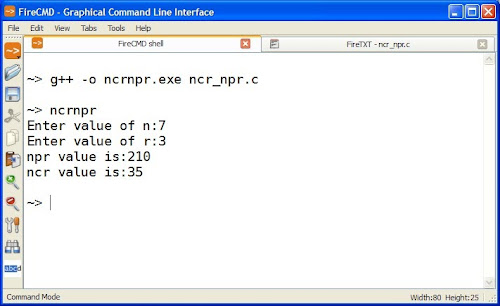C program to find nCr and nPr (Combinations and Permutations)

This is a C language program code to find nCr (Combinations) and nPr (Permutations). Note that the program uses following formulas to find nCr and nPr.

The permutations formula is (P(n,r) = n! / (n - r)!). The combinations formula is (C(n,r) = n! / r! (n - r)!). The exclamation mark in the formula suggests factorial. To avoid repetitiveness, we are using a function to calculate factorial.

/* Program to calculate nCr and nPr by reading values of n and r from user*/
#include <stdio.h>
#include <conio.h>
long int factorial (int x);

int main ()
{
int n,r;
long int ncr,npr;
printf ("Enter value of n:");
scanf ("%ld",&n);
printf ("Enter value of r:");
scanf ("%ld",&r);
npr = factorial(n)/factorial(n-r);
ncr = npr / factorial(r);
printf ("npr value is:%ld\n",npr);
printf ("ncr value is:%ld\n",ncr);
getch ();
return 0;
}

/* Function to calculate factorial */
long int factorial (int x)
{
int i,f=1;
for (i=2 ; i<=x ; i++)
f = f * i;
return (f);
}

OutputNote: I have used FireCMD shell to compile the program.